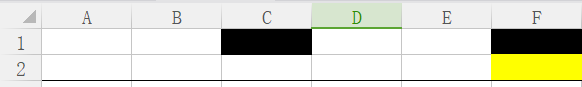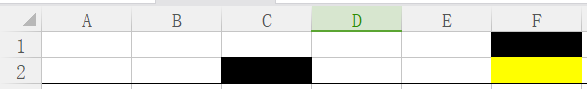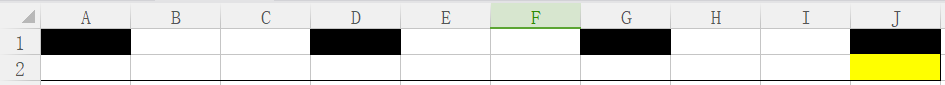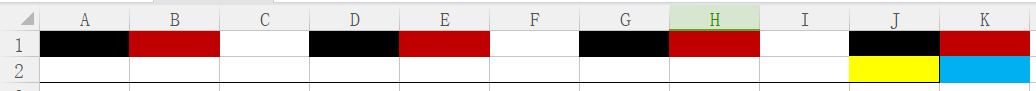# 单调队列优化的背包问题

## 01背包问题

f[i][v]表示前i件物品恰放入一个容量为v的背包可以获得的最大价值。则其状态转移方程便是：

f[i][v]=max{f[i-1][v],f[i-1][v-c[i]]+w[i]}。## 完全背包问题

f[i][v]=max{f[i-1][v],f[i][v-c[i]]+w[i]}# 多重背包问题 （正文）

https://blog.csdn.net/hebtu666/article/details/82720880//输入
int n;
int W;
int w[MAX_N];
int v[MAX_N];
int m[MAX_N];

int dp[MAX_N+1];//压空间，本知识参考https://blog.csdn.net/hebtu666/article/details/79964233
int deq[MAX_N+1];//双端队列，保存下标
int deqv[MAX_N+1];//双端队列，保存值void solve()
{
for(int i=0;i<n;i++)//参考过那个网址第二题应该懂
{
for(int a=0;a<w[i];a++)//把每个分组都打一遍
{
int s=0;//初始化双端队列头尾
int t=0;
for(int j=0;j*w[i]+a<=W;j++)//每组第j个元素
{
int val=dp[j*w[i]+a]-j*v[i];
while(s<t && deqv[t-1]<=val)//直到不改变单调性
t--;
deq[t]=j;
deqv[t]=val;
t++;
//利用队头求出dp
dp[j*w[i]+a]=deqv[s]+j*v[i];
if(deq[s]==j-m[i])s++;//检查过期
}
}
}
}

04-07
07-0404-1855
02-082647
10-155351
10-2869
03-17550
06-27191
04-131139
09-05470